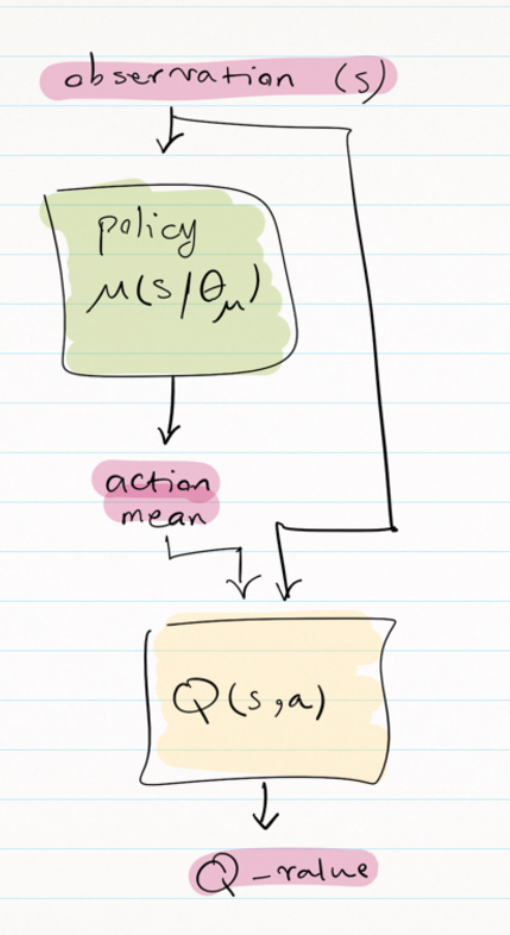# RL Series – DDPG

Original article can be found here (source): Deep Learning on MediumThis is part of my RL-series posts.

This algorithm is from the “Continuous Control with Deep Reinforcement Learning” paper and uses the ideas from deep q-learning in the continuous action domain and is a model-free method based on the deterministic policy gradient.

In Deterministic Policy Gradient (DPG), for each state, we have one clearly defined action to take. But in Stochastic Gradient Descent, we have a distribution over actions and sample from that distribution to get the action. In another term, in stochastic policy gradient, we have a distribution with mean and variance and we draw a sample from that as an action. When we reduce the variance to zero, the policy will be deterministic.

When the action space is discrete, such as q-learning, we get the max over q-values of all actions and select the best action. But in continuous action spaces, you cannot apply q-learning directly, because in continuous spaces finding the greedy policy requires optimization of a_t at every time-step and would be too slow for large networks and continuous action spaces. Based on the proposed equation in the reference paper, here we approximate max Q(s, a) over actions with Q(a, µ(s)).

In DDPG, they used function approximators, neural nets, for both action-value function Q and deterministic policy function µ. In addition, DDPG uses some techniques for stabilizing training, such as updating the target networks using soft updating for both μ and Q. It also uses batch normalization layers, noise for exploration, and a replay buffer to break temporal correlations.

This algorithm is an actor-critic method and the network structure is as follows:

First, the policy network gets the state and outputs the action mean vector. This will be a vector of mean values for different actions. For example, in a self-driving car, there are two continuous actions: steering and acceleration&braking (one continuous value between -x to x, the negative values are for braking and positive values are for acceleration). So we will have two mean for these two actions. To consider exploration, we can use Ornstein-Uhlenbeck or normal noise and add it to the action mean vector in the training phase. In the test phase, we can use the mean vector directly without any added noise.

Finally, the sudo code for DDPG is as follows: